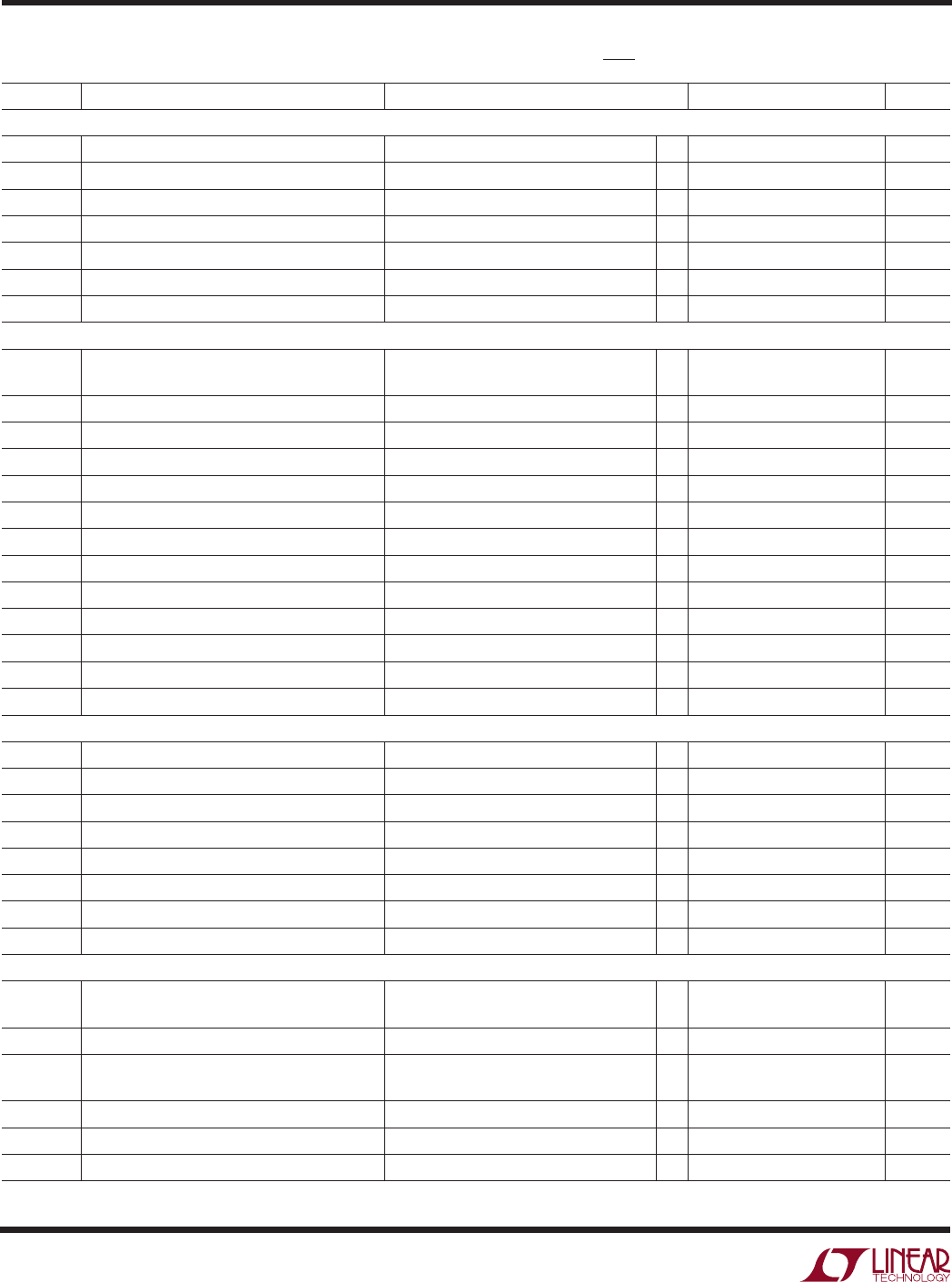# Datasheet4
LTC2846
sn2846 2846fs
The denotes specifications which apply over the full operating
temperature range, otherwise specifications are at T
A
= 25°C. V
CC
= 5V, V
IN
= 3.3V, V
SHDN
= V
IN
, unless otherwise noted. (Notes 2, 3)
ELECTRICAL CHARACTERISTICS
SYMBOL PARAMETER CONDITIONS MIN TYP MAX UNITS
V
TH
Input Threshold Voltage 7V V
CM
7V 0.2 0.2 V
V
TH
Input Hysteresis 7V V
CM
7V 15 40 mV
R
IN
Input Impedance 7V V
CM
7V (Figure 3) 100 103
t
r
, t
f
Rise or Fall Time C
L
= 50pF (Figures 4, 14) 15 ns
t
PLH
Input to Output Rising C
L
= 50pF (Figures 4, 14) 50 90 ns
t
PHL
Input to Output Falling C
L
= 50pF (Figures 4, 14) 50 90 ns
t Input to Output Difference, t
PLH
– t
PHL
C
L
= 50pF (Figures 4, 14) 0425 ns
V.35 Driver
V
OD
Differential Output Voltage Open Circuit, R
L
= 1.95k (Figure 5) ±1.2 V
CM
4V (Figure 6) ±0.44 ±0.55 ±0.66 V
V
OA
, V
OB
Single-Ended Output Voltage Open Circuit, R
L
= 1.95k (Figure 5) ±1.2 V
V
OC
Transmitter Output Offset R
L
= 50 (Figure 5) ±0.6 V
I
OH
Transmitter Output High Current V
A
,
V
B
= 0V –9 11 –13 mA
I
OL
Transmitter Output Low Current V
A
,
V
B
= 0V 91113 mA
I
OZ
Transmitter Output Leakage Current V
A
and V
B
0.25V ±1 ±100 µA
R
OD
Transmitter Differential Mode Impedance 50 100 150
R
OC
Transmitter Common Mode Impedance 2V V
CM
2V (Figure 7) 135 150 165
t
r
, t
f
Rise or Fall Time (Figures 8, 13) 5 ns
t
PLH
Input to Output (Figures 8, 13) 15 35 65 ns
t
PHL
Input to Output (Figures 8, 13) 15 35 65 ns
t Input to Output Difference, t
PLH
– t
PHL
(Figures 8, 13) 016 ns
t
SKEW
Output to Output Skew (Figures 8, 13) 4 ns
V
TH
Differential Receiver Input Threshold Voltage 2V V
CM
2V (Figure 9) 0.2 0.2 V
V
TH
CM
2V (Figure 9) 15 40 mV
R
ID
Receiver Differential Mode Impedance 2V V
CM
2V 90 103 110
R
IC
Receiver Common Mode Impedance 2V V
CM
2V (Figure 10) 135 150 165
t
r
, t
f
Rise or Fall Time C
L
= 50pF (Figures 4, 14) 15 ns
t
PLH
Input to Output C
L
= 50pF (Figures 4, 14) 50 90 ns
t
PHL
Input to Output C
L
= 50pF (Figures 4, 14) 50 90 ns
t Input to Output Difference, t
PLH
– t
PHL
C
L
= 50pF (Figures 4, 14) 0425 ns
V.28 Driver
V
O
Output Voltage Open Circuit ±10 V
R
L
= 3k (Figure 11) ±5 ±8.5 V
I
SS
Short-Circuit Current V
OUT
= GND ±150 mA
R
OZ
Power-Off Resistance 2V < V
O
< 2V, Power Off 300
or No-Cable Mode
SR Slew Rate R
L
= 7k, C
L
= 0 (Figures 11, 15) 430V/µs
t
PLH
Input to Output R
L
= 3k, C
L
= 2500pF (Figures 11, 15) 1.5 2.5 µs
t
PHL
Input to Output R
L
= 3k, C
L
= 2500pF (Figures 11, 15) 1.5 2.5 µs